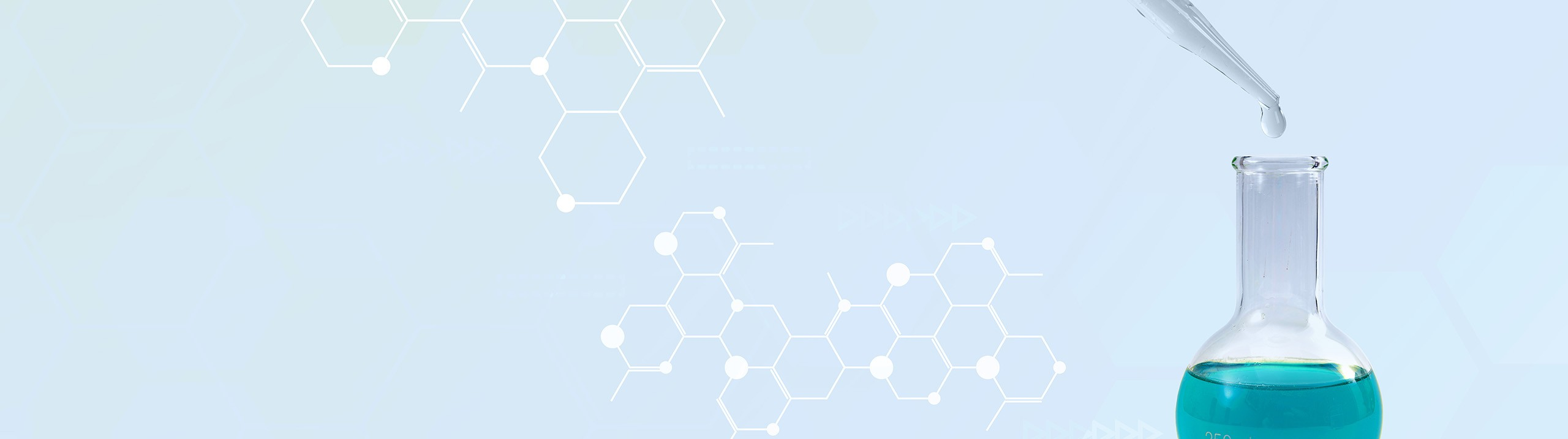## Analytical Chemistry

Learn the toughest concepts covered in your Analytical Chemistry class with step-by-step video tutorials and practice problems.

7. Activity and the Systematic Treatment of Equilibrium

# Ionic Strength of Soluble Salts

Ionic strength represents the measurement of all the ions in the aqueous solution.

Ionic Strength
1
concept

## Ionic Strength5m
Play a video:
2
example

## Ionic Strength2m
Play a video:
So here we have to calculate the ionic strength of the following ionic compound. So here this compound is copper two sulfate. It breaks up into copper two ion plus the sulfide ion. Now how do I know that copper has a plus two charge? Well you need to remember your poly atomic ion S. +03. Here is sulfide ion, right? So that's the formula sulfide ion. If the overall compound is neutral and it's a 1 to 1 relationship, one comfort of one cell fight then if this is two minus, comprehends to be two plus the charges cancel out and have a neutral ionic compound at the end. So right now we have a 1 to 1 relationship of the ions. If the concentration for the entire ionic compound is 0.10 moller then each ion produced will have that same concentration. So here this is 0.10 moller and this is 0.10 moller. Now we're gonna say that our ionic strength equals half. So the concentration of the first ion which is .010 Times its charge squared. So plus two squared plus the concentration of the second ion times its charge squared. So when we do all that, we'll get an answer of .04 for the ionic strength of 0.10 moller, copper two sulfate. So just follow the steps that we did if there were multiple ions. So let's say that there were two coppers for some reason that we'd have to multiply the concentration by two because we have to take into account all the copper ions with the overall concentrations that they have. But here, since it's a 1 to 1, the initial concentration I have for the compound matches the concentrations for the ions provided. Later on, we'll continue more questions that deal with determining the ionic strength of an overall ionic compound. So again, make sure you remember your poly atomic ions and how to correctly classify uh the relationships between the ions and their charges involved.
Ionic Strength Calculations
3
example

## Ionic Strength Calculations2m
Play a video:
So just as we stated in the previous videos when figuring out the ionic strength for any ionic compound, it's best to break it up into its ions to see how many ions we have of each one. So we can figure out their concentrations. So here we have aluminum carbonate, aluminum carbonate breaks up into two aluminum ions Plus three carbonate ions. The concentration of the entire ionic compound is this but the number of ions of each type determines their overall concentration. Here we have two aluminums, so it be two times the original concentration. So the overall concentration of aluminum ions would be .060 moller. Here we have three carbonates, so that's three times the original concentration. So the overall concentration of carbonate is .090 moller. Now we use the formula to determine ionic strength. So ionic strength equals half. Remember it's the summation of we're gonna say the concentration of the ion times its charge squared plus concentration of the next ion times its charge squared. So that'll be half the concentration of my aluminum ion is 0.60 moller times its charge squared. So plus three squared. And then plus the concentration of bicarbonate ion, which is .090 moller Times its charge squared which is -2 squared. So here, if you work out what's in the brackets correctly, you'll get half of .90. So that would mean that my on ionic strength here would be .45. Now that you've seen this example, Try the next one and this one we're asked to figure out the total ionic strength of this solution. So here we have two different ionic compounds mixing together. What effect would that have on the concentration of each ion present to determine the final ionic strength? Try it out for yourself and then come back and see how I approach the same example to question.
4
example

## Ionic Strength Calculations3m
Play a video: